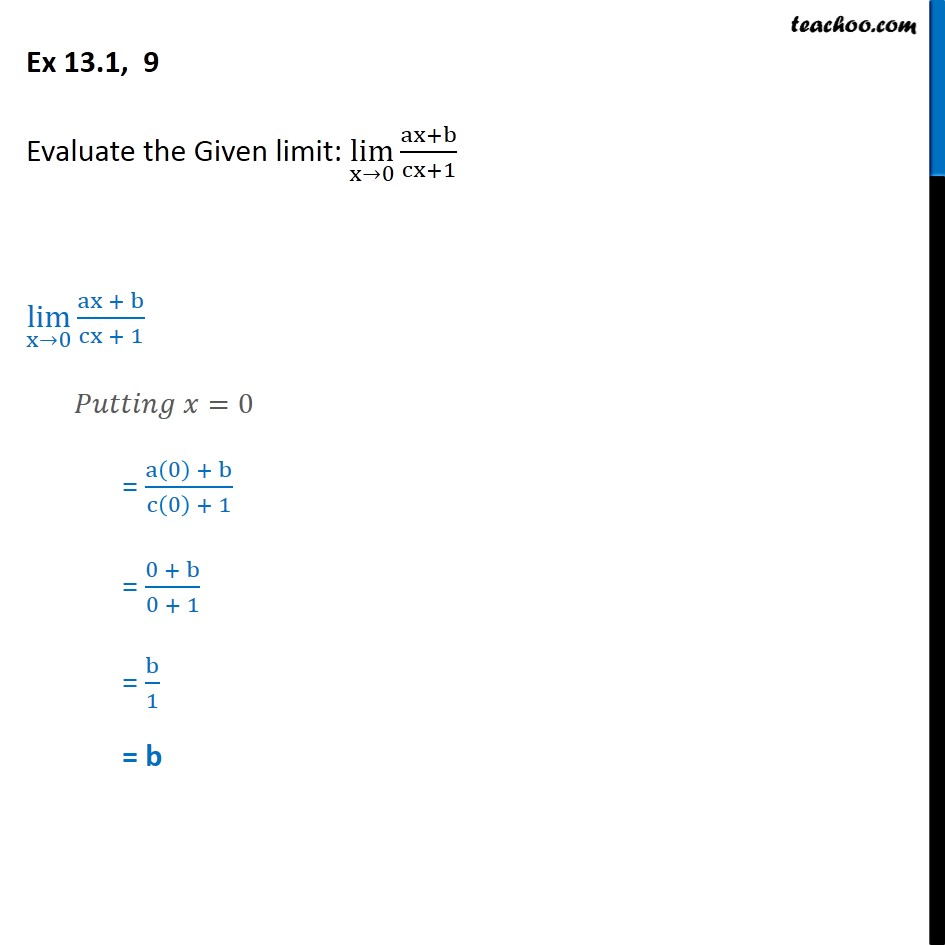1. Chapter 13 Class 11 Limits and Derivatives (Term 1 and Term 2)
2. Serial order wise
3. Ex 13.1 (Term 1)

Transcript

Ex 13.1, 9 - Chapter 13 Class 11 Limits and Derivatives - NCERT Evaluate the Given limit: lim (x→0) (ax+b)/(cx+1) lim (x→0) (ax + b)/(cx + 1) Putting x = 0 = (a(0) + b)/(c(0) + 1) = (0 + b)/(0 + 1) = b/1 = b